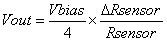eCircuit  Center

### SENSOR BRIDGE

CIRCUITSuppose you have a sensor that converts a physical phenomenon like temperature or pressure into a resistance change. How do you convert the resistance change into a useful voltage you can measure?

SENSOR BRIDGE

A sensor bridge gives you a differential output voltage given a sensor's resistance change. The resistive sensor XSENSOR is placed in one of four branches of the bridge. XSENSOR is a subcircuit that models a voltage controlled resistor. Resistors R1, R2, and R4 are chosen to be equal to the nominal value of XSENSOR.

The voltage at node 2 is developed from voltage divider R1 and XSENSOR. The voltage at node 3 comes from voltage divider R2 and R4. When all resistors are equal, the voltage at nodes 2 and 3 are equal. Therefore, the difference between 2 and 3 is zero. However, a resistance change of XSENSOR unbalances the two voltage dividers producing a useful voltage change between 2 and 3.

TEMPERATURE SENSOR

Suppose you’ve selected a temperature sensor whose resistance is 1k ohms at 25 deg C and changes ΔRsensor = +/- 100 ohms at temperatures 50 and 0 deg C.

T deg C          R (ohms)
0                       900
25                    1000
50                    1100

Choose  RB1, RB2 and RB4 = 1k to produce 0 volts output at 25 deg C. But, what kind of voltage change can you expect from ΔRsensor=100 ohm? For small resistance changes the differential output can be approximated byCIRCUIT ANALYSIS  For Rsensor = 1k,  ΔRsensor=100 and VBIAS = 10 V, calculate the output voltage swing to be Vout = (10/4) x (100 / 1k) = 0.25 V. The voltage ramp from VCONT controls the resistance of the XSENSOR changing it from 900 to 1100 ohms over 10ms. Run a simulation and plot the voltage at V(2) and V(3). Do you see V(2) producing the expected +/- 0.25V change from the 5V bias point?

SENSOR BRIDGE KEY FUNCTION

What’s interesting is that the small voltage change is centered around 5V! This doesn’t help us especially if we want to amplify this signal at a later stage. We would end up amplifying the 5V too - saturating the amplifier stage!

It would be great if we could subtract off the 5V to produce a more useful output. That’s where the other leg of the bridge comes in. Note that V(3) is conveniently sitting at 5V. So now by taking the differential voltage at Vout, you get a much more useful result. View the difference between nodes 2 and 3 by the plotting the variable V(2,3) to see the +/- 0.25V output centered around 0V. ( Here is a great application for a differential or instrumentation amplifier to measure the difference voltage V(2) - V(3). )

NON-LINEAR OPERATION

What are the limitations of a single sensor in a four legged bridge? The output voltage versus resistance becomes non-linear for large resistance changes.

CIRCUIT INSIGHT   Increase the resistance change from +/-100 to a greater value like +/-500 ohms, for example. You can do this by changing the control voltage to look like

VCONT 10 0  PWL(0MS 0.5   10MS 1.5)

VCONT now generates a ramp from 0.5 to 1.5V that effectively sweeps the nominal 1000 resistor of XSENSOR from 500 to 1500 ohms. Run a simulation and plot V(2,3). Can you see a bend in the output voltage?

SIMULATION NOTE

Although SPICE does not directly have a model for voltage controlled resistor, you can put one in your circuit using the subcircuit XSENSOR. Change the value of 1K in the VALUE expression to the nominal resistance value you desire. This resistance will appear at the output terminals (1) and (2) when the control voltage at nodes (4) and (5) is 1.0V. The output resistance will then scale according to the control voltage value. ( For more info, see Voltage Controlled Resistor. )

SPICE FILE

Download the file or copy this netlist into a text file with the *.cir extention.

```SBRIDGER.CIR - INSTRUMENTATION BRIDGE
*
VBIAS	1	0	DC	10
*
* INSTRUMENTATION BRIDGE
RB2	1	3	1K
RB4	3	0	1K
RB1	1	2	1K
*
* RESISTIVE SENSOR (VOLTAGE CONTROLLED)
XSENSOR	2 0 10 0 V_CONT_R
*
* CONTROL VOLTAGE
VCONT	10 	0	PWL(0MS 0.9  10MS 1.1)
RD1	10 	0	1G
*
* SUBCIRCUIT FOR VOLTAGE CONTROLLED RESISTOR
*	RESISTOR - 1,2     CONTROL - 4,5
*
.SUBCKT V_CONT_R   1  2  4  5
ERES	1       3       VALUE = { I(VSENSE)*1K*V(4,5) }
VSENSE	3	2	DC	0
.ENDS
*
* ANALYSIS
.TRAN 1MS 10MS
* VIEW RESULTS
.PLOT	TRAN V(2) V(3)
.PRINT	TRAN V(2) V(3)
.PROBE
.END```

Top↑